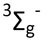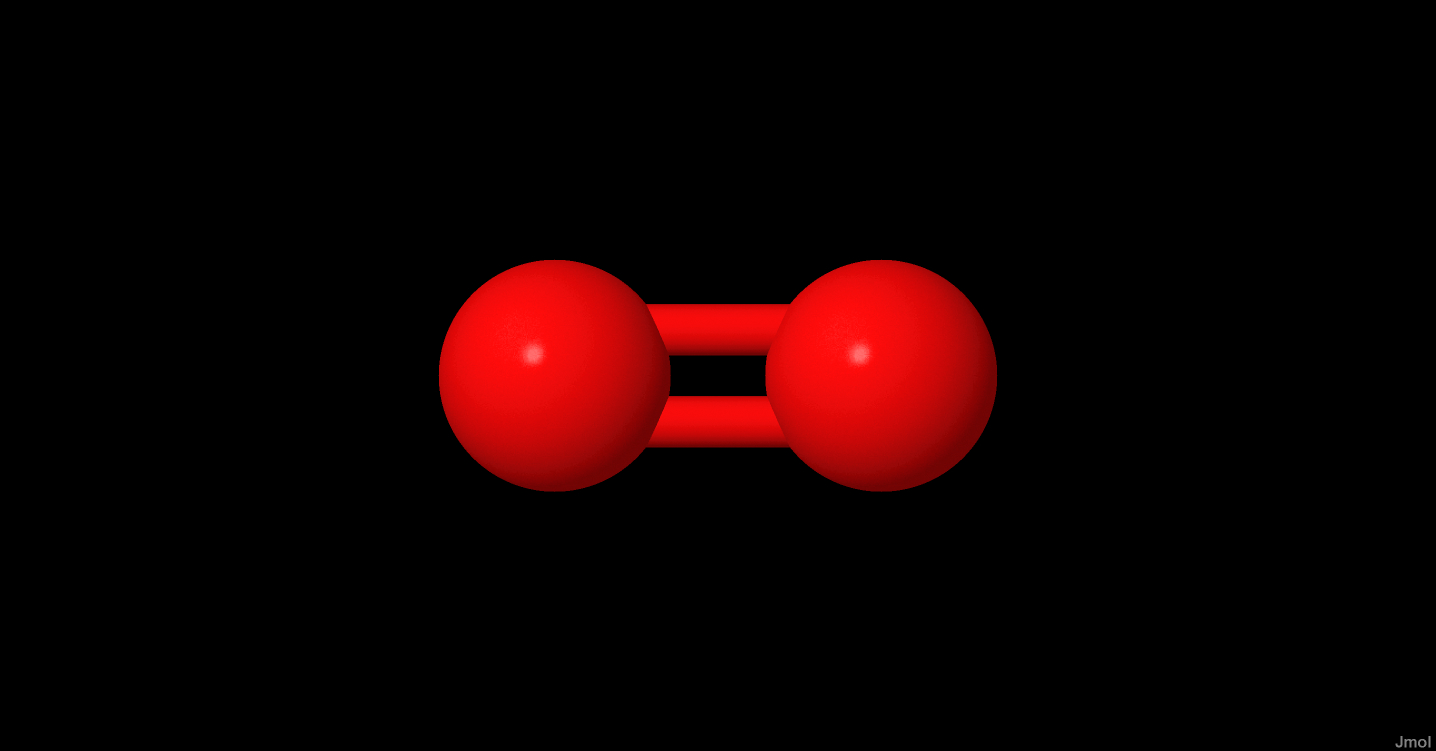# O2

Click on the   icon info to get details.

Species data
 Common Formula O2 Stoichiometric Formula O2 Name Molecular oxygen Mass 31.98983 a.m.u Charge 0 CAS 7782-44-7 Inchi InChI=1S/O2/c1-2 InchiKey MYMOFIZGZYHOMD-UHFFFAOYSA-N State Ground StateISM Abundance
log10 Abundance Reference Source Name Source Type Link
Polarizability
 Definition: total Value (Å3): 1.562 Method: Measurements Origin: Database : NIST COMPUTATIONAL CHEMISTRY COMPARISON AND BENCHMARK DATABASE Reference:
Dipole moment
 Value (D): 0 Method: N/A Origin: Database : NIST COMPUTATIONAL CHEMISTRY COMPARISON AND BENCHMARK DATABASE Reference:
Enthalpy of formation
 T (K): 298 Value (kJ.mol-1) : 0 Method: N/A Origin: Other database Reference: T (K): 0 Value (kJ.mol-1) : 0 Method: N/A Origin: Other database Reference:
Desorption energy
 Emean (K): 1200 ±360 E min (K): 0 E max (K): 0 Pre-exponential factor (s-1): 0.00E+0 Method: Calculations Origin: Bibliography Reference: Wakelam, V. et al. ;2017;ArXiv e-prints;, Type of surface: H2O Description: To estimate the unknown binding energies (for most of the radicals for example), we have developed a model founded on the stabilization energy of the complex between the various species and one water molecule. Then, we assume that the binding energy of the species with ASW is proportional to the energy of interaction between this species and one water molecule. To determine the proportionality coefficients, we fit the dependency of the experimental binding energies versus the calculated energies of the complexes for 16 stable molecules. Uncertainties in ED is estimated to be 30%. The preexponential factor is to be computed using the Hasegawa et al. (1992) approximation. Evaluation: Emean (K): 1107 E min (K): E max (K): Pre-exponential factor (s-1): 5.98E+14 Method: Reviews and Evaluations Origin: Bibliography Reference: Minissale, Marco et al. ;2022;ACS Earth and Space Chemistry;, Type of surface: Compact Amorphous Solid Water Description: Uncertainties are discussed in Minissale et al. (2022) Evaluation: Emean (K): 1522 E min (K): E max (K): Pre-exponential factor (s-1): 5.98E+14 Method: Reviews and Evaluations Origin: Bibliography Reference: Minissale, Marco et al. ;2022;ACS Earth and Space Chemistry;, Type of surface: Highly-Oriented Pyrolytic Graphite Description: Uncertainties are discussed in Minissale et al. (2022) Evaluation: Emean (K): 1385 E min (K): E max (K): Pre-exponential factor (s-1): 5.98E+14 Method: Reviews and Evaluations Origin: Bibliography Reference: Minissale, Marco et al. ;2022;ACS Earth and Space Chemistry;, Type of surface: SiOx Description: Uncertainties are discussed in Minissale et al. (2022) Evaluation: Emean (K): 1000 E min (K): 0 E max (K): 0 Pre-exponential factor (s-1): 0.00E+0 Method: Estimation Origin: Other database Reference: Type of surface: H2O Description: This binding energy was listed in the original OSU gas-grain code from Eric Herbst group in 2006. Herma Cuppen's suggestion The pre-exponential factor is not given. It can be computed using the formula given in Hasegawa et al. (1992). Evaluation:
Diffusion energy
No data